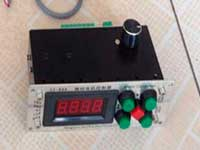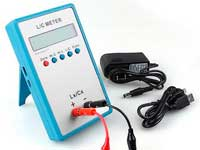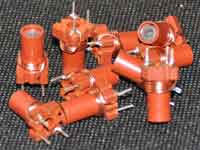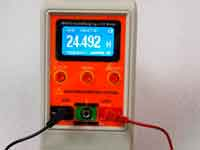One turn loop inductance calculation - 5.0 out of 5 based on 1 vote
Rating 5.00 (1 Vote)

Single circular loop inductorInductance realized in the form of a single circular loop, in other words in the form of a single turn, most often used in the UHF band. Due to the lack of proximity effect, has a fairly high Q-factor, depending on wire diameter and a small self-capacitance. Also such inductors we can see as magnetic detectors.

The single-turn loop can be calculated by numerical methods using J.C. Maxwell equation:• E(k) is the complete elliptic integral of the first kind
• K(k) is the complete elliptic integral of the second kind
• r = D/2 is the radius of the loop (m)
• a = d/2 is the radius of the wire cross-section, half of the wire diameter (m)

and the parameter k is calculated as follows:However, in the Coil32 calculation of the loop inductance is carried by a simple empirical formula, which was first brought by F. W. Grover in 1946. In this case, there is no sense to apply more complicated algorithm of calculation, because the accuracy is quite sufficient for practical purposes, when D/d > 5 to about 1%. The numerical algorithm of the program is based on the method of successive approximations to reach a required inductance. The maximum possible diameter of the loop is taken as 10 m. If the calculation is obtained by a larger diameter value, the program displays the message: "Coil cannot be realized". In this case, you must choose another form of coils, for example, singlelayer.

One loop inductance formula:• L - inductance (µH)
• D - diameter of loop (mm)
• d - diameter of the wire (mm)

The diameter of the loop is from center to center of the wire as on a figure above.

Сomments from anonymous guests are enabled with moderation.

Coil Winding MachineUS \$187.53
The system consists of individual components including a controller, a stepping motor, a foot switch, power adapter and output shaft. This design makes it very easy for you to integrate the system into your own project.

LC Meter LC200AUS \$37.99
Measuring Capacitance Range:
0.01 pF - 100mF
Measuring Inductance Range:
0.001 uH - 100H
Measurement accuracy:1%.

RF InductorsUS \$6.43
Variable Ferrite Core Inductor coil set 3.5Turns 25-100MHZ

Auto Ranging LCR MeterUS \$63.07
Meter can measure 0.00pF to 100.00mF capacitance, 0.000uH to 100.00H inductance and 0.00? to 10.000M? resistance. Very suitable for small value capacitor and inductor measurement.